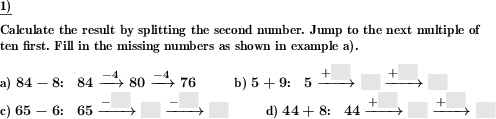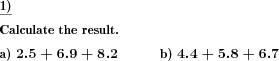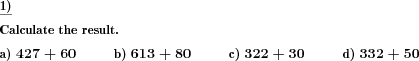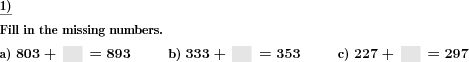Custom math worksheets at your fingertips# Details for problem "Determine shapes with same shaded fraction"

Quickname: 2211

Elementary School, Primary School, Middle School.

## Summary

In a series of shapes find the two shapes that represent the same fraction.

## Example## Description

A series of geometric shapes is presented. Each shape representing a fraction is divided into equal parts. The number of parts corresponds to the denominator of the fraction. Some of the parts are marked in color. The number of marked parts corresponds to the numerator.

Exactly two figures represent the same fraction, i.e. the value of the fraction is equal. It may be necessary to reduce fractions to determine equality.

The number of shapes per problem can be set, as well as the number of problems.

## Free worksheets and solution sheets with answers for download

Download free printable worksheets for this math problem here. The worksheet contains the problems only, the solution sheet includes the answers. Just click on the respective link.

•Worksheet 1Solution sheet with answers
•Worksheet 2Solution sheet with answers
•Worksheet 3Solution sheet with answers

If you can not see the solution sheets for download, they may be filtered out by an ad blocker that you may have installed. If this is the case, please allow ads for this page and reload the page. The solution sheets will then reappear.

• Do these sample worksheets do not really fit?
• Do you need more math worksheets, with a different level of difficulty?
• Would you like to combine different problems on a worksheet and adjust them to your needs?
• As a teacher, you can put together your own worksheets using the automatically generated math problems provided.
With a free initial credit, you can start creating your own math worksheets in a few minutes.

You can try it for free! Register here, to create custom worksheets now!

## Customization options for this problem

Parameter
Possible values
Number of problems
1, 2
Number of shapes
3, 4, 5, 6
All shapes share same denominator
Yes, No

## Similar problems

Remark
Description
Name and direct link
Shade fractions of given shapes as indicated
An image of s subdivided shape is shown. Shade according to given fraction.
Shade a series of shapes to show the same fractions
Shade n/m, 2n/2m, 3n/3m and so on of shapes with increasing number of parts.

## Other types of problems that appear on worksheets with this problem:

Relevance
Name
Description
Quickname
Example
****
Add and subtract spliting one digit number
Addition and subtraction by splitting one digit number****
Numbers with or without decimal places have to be added.****
Add multiples of powers of ten
Multiples of powers of ten added to natural numbers.****
Addition of powers of ten, fill blanks
What multiple of a power of ten has been added?You may pass on the worksheets offered for download on this page to others, but not modify or sell them.